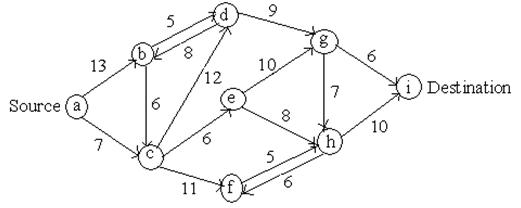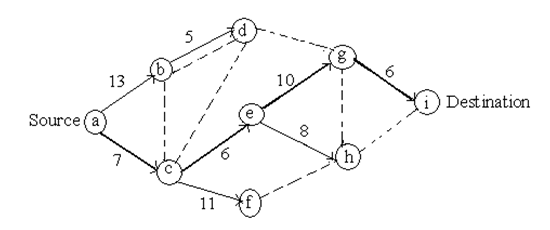#### Shortest Route Problem Assignment Help

Shortest Route Problem

The criterion of this technique is to determine the shortest distance between two nodes with least cost.

Get the shortest pathSteps to find Shortest Route Problem

 n Solved nodes directly connected to unsolved nodes Closest connected unsolved node Total distance involved nth nearest node Minimum distance Last connection 1 a c 7 c 7 a-c 2 a c b e 13 7+6 =13 b e 13 13 a-b c-e 3 b c e d f h 13+5 =18 7+11 =18 13+8 =21 d f - 18 18 - b-d c-f - 4 e d f h g h 13+8 =21 18+9 =27 18+5 =23 h - - 21 - - e-h - - 5 e h d g i g 13+10 =23 21+10 =31 18+9 =27 g - - 23 - - e-g - - 6 g h i i 23+6 =29 21+10 =31 i - 29 - g-i -The shortest path from a to i is a → c →e →g → i

Distance = 7 + 6 + 10 + 6 = 29 units

Shortest Route Problem Assignment Help – Homework Help

Finding answers for shortest route problems are quite interesting and in solving question, a student needs to clear steps to follow shortest path. Sometimes student needs an expert help for solving operation research problems, We at www.tutorsglobe.com offer Shortest Route Problem assignment help, Shortest Route Problem homework help, answering question for shortest path and operation research homework help. We offer email based service for homework help and assignment help, easy for students to get assistance through live tutors.